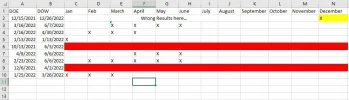# Determine if a month falls between two dates.

R

#### Robert R

Hello, I have been tasked with trying to do the following

Column A - Date
column B -Date

A will always be earlier than B

columns C-N will be labeled months Jan-Dec

What I need to do is determine if the date between column A and B includes
the given month in column C - N and return a value of X.

ie...
A B C D E F
Jan Feb Mar Apr
1/1/10 3/12/10 X X X blank

I can then count the X values.

Any help would be greatly appreciated.

T

#### T. Valko

Column A - Date
column B -Date
A will always be earlier than B

Are the dates all within the same year? Will you ever have dates like this:

A2 = 6/25/2010
B2 = 1/12/2011

F

#### Fred Smith

I would do this in two steps:
1. Put actual dates in C1 to N1. Use a format of "mmm" which will display
the 3-character month name.
2. To get the X's, use:
=if(and(month(c\$1)>=month(\$a2),month(c\$1)<=month(\$b2)),"X","")

Regards,
Fred

R

#### Robert R

T. Valko,

Actually, the dates should always be in the same year

T

#### T. Valko

Fred's suggestion will work.

Here's another method that is basically the same but doesn't require the

C1:N1 = month names as text entries: Jan, Feb, Mar, ... Dec

A2:B2 = some dates

Enter this formula in C2 and copy across to N2:

=IF(AND(COLUMNS(\$C2:C2)>=MONTH(\$A2),COLUMNS(\$C2:C2)<=MONTH(\$B2)),"X","")

#### exceluser01

This is an ancient thread but does anyone know how to make this formula work for dates that span into the next year?

ie

A2 = 9/15/2021
B2 = 4/27/2022

The example above works as long as the dates are in the same year... I need it for education where the school year runs from Aug - June... Any help would be appreciated.

See the attached file for a sample of what the formula shown above produces.

#### Attachments

•sample.JPG
59.7 KB · Views: 4